2019-11-21 18:18:19 CSDNedu 阅读数 356
• ###### python数据分析与机器学习实战【2019新版】

购买课程后，请扫码进入学习群，获取唐宇迪老师答疑 Python数据分析与机器学习实战教程，该课程精心挑选真实的数据集为案例，通过python数据科学库numpy,pandas,matplot结合机器学习库scikit-learn完成一些列的机器学习案例。课程以实战为基础，所有课时都结合代码演示如何使用这些python库来完成一个真实的数据案例。算法与项目相结合，选择经典kaggle项目，从数据预处理开始一步步代码实战带大家入门机器学习。学完该课程即可： 1.掌握Python数据科学工具包，包括矩阵数据处理与可视化展示。 2.掌握机器学习算法原理推导，从数学上理解算法是怎么来的以及其中涉及的细节。 3.掌握每一个算法所涉及的参数，详解其中每一步对结果的影响。 4.熟练使用Python进行建模实战，基于真实数据集展开分析，一步步完成整个建模实战任务。

93869 人正在学习 去看看 唐宇迪## 近日，华为20亿奖励员工的新闻频频刷屏。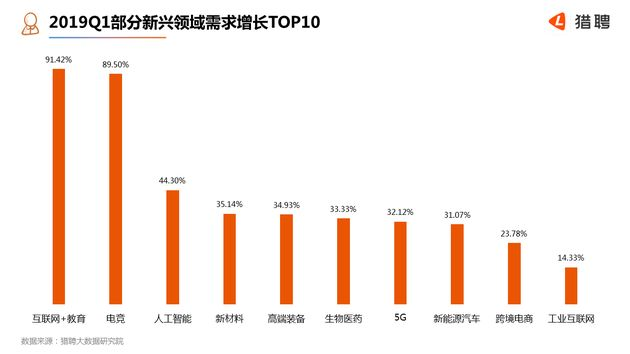BOSS直聘研究院的数据显示，2018年，所有要求掌握AI、算法、智能识别等相关技能的岗位，AI年度薪资总和规模至少达到15亿元，较2017年增长5.8倍！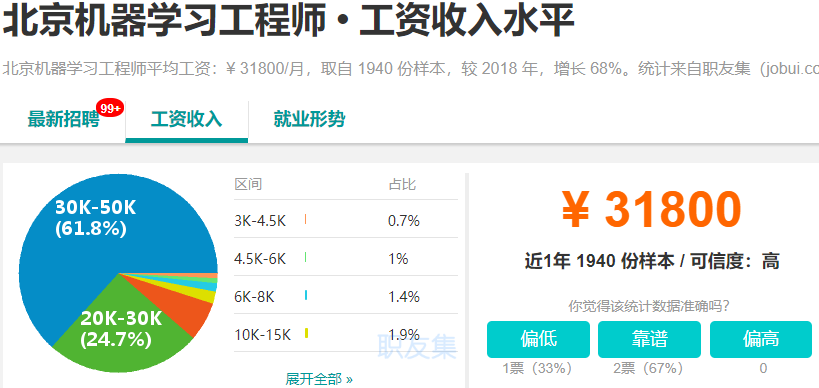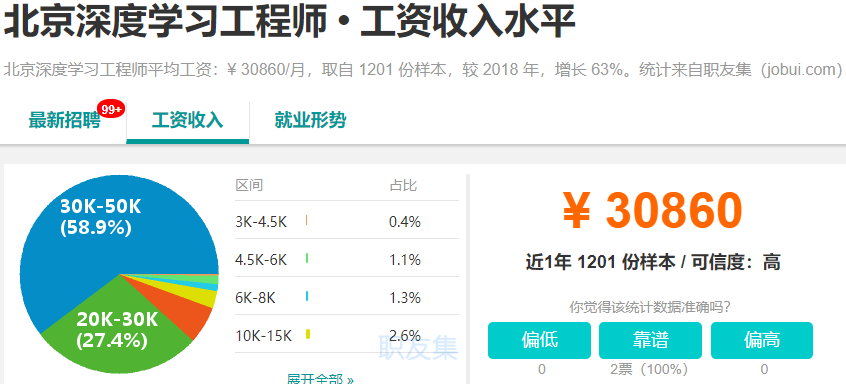CSDN精心打磨了一套针对转型/进阶同学的+书复合课程《决胜AI—机器学习+深度学习系列实战200讲》

| 图书邮寄 | 视频精讲 | 项目实战 | 社群答疑 |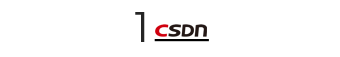97%的好评，4万程序员在看

## 课程亮点：系统学习人工智能

1、机器学习+深度学习核心内容面面俱到

2、基于Python主流趋势持续更新，一劳永逸

3、书籍+线上复合型场景，多样化学习方式，轻松不累

4、录播课程随到随学，社群答疑解决学习难题

5、面向企业需求，零基础及进阶人工智能工程师准备

6、提供项目实战资料及源码，轻松复现

## #核心课程内容摘录#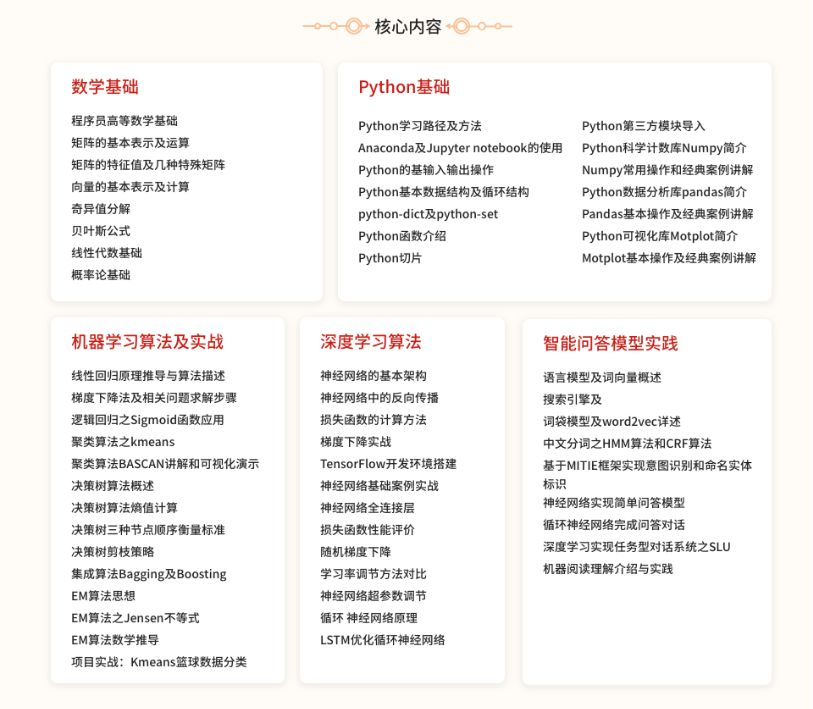## #视频课+图书教材#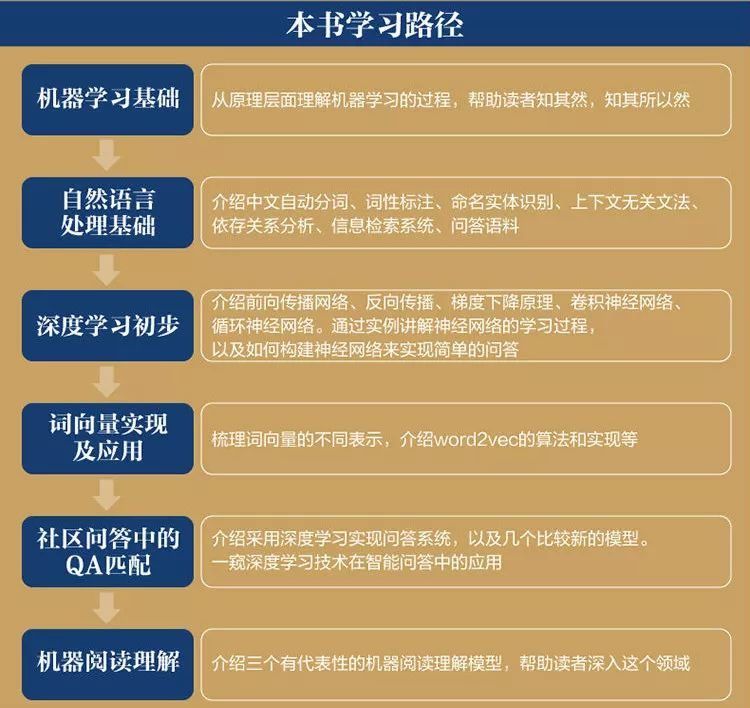| 图书邮寄 | 视频精讲 | 项目实战 | 社群答疑 |## #你将收获#

• 1本实体图书教材，京东畅销书《智能问答与深度学习》含邮寄

• 5大模块，涉及机器学习算法、神经网络、中文分词等AI热点领域，成为AI技术高手

• 200讲机器学习+深度学习系列实战，将理论与实战相结合，做到真正的学以致用

• 专属微信社群，随时答疑，将AI彻底掌握

| 图书邮寄 | 视频精讲 | 项目实战 | 社群答疑 |（回复数字2入群）k聚类机器学习实战 相关内容

2015-07-28 20:55:50 u010412719 阅读数 5517
• ###### python数据分析与机器学习实战【2019新版】

购买课程后，请扫码进入学习群，获取唐宇迪老师答疑 Python数据分析与机器学习实战教程，该课程精心挑选真实的数据集为案例，通过python数据科学库numpy,pandas,matplot结合机器学习库scikit-learn完成一些列的机器学习案例。课程以实战为基础，所有课时都结合代码演示如何使用这些python库来完成一个真实的数据案例。算法与项目相结合，选择经典kaggle项目，从数据预处理开始一步步代码实战带大家入门机器学习。学完该课程即可： 1.掌握Python数据科学工具包，包括矩阵数据处理与可视化展示。 2.掌握机器学习算法原理推导，从数学上理解算法是怎么来的以及其中涉及的细节。 3.掌握每一个算法所涉及的参数，详解其中每一步对结果的影响。 4.熟练使用Python进行建模实战，基于真实数据集展开分析，一步步完成整个建模实战任务。

93869 人正在学习 去看看 唐宇迪

## 《机器学习实战》之K-均值聚类算法的python实现

### 基本K-均值聚类算法

对数据集中的每一个数据点
对每一个质心
计算质心与数据点的距离
将数据点分配到距离最近的簇
对每一个簇，计算簇中所有点的均值，并将均值作为质心

python实现代码如下：注释基本上写的相当相当详细，由于自己还是python的初学者，觉得注释过多不方便看，还请大家谅解，有错误希望大家指正。

pip install numpy
pip install matplotlib

KMeans.py文件

``````from numpy import *
import time
import matplotlib.pyplot as plt

# calculate Euclidean distance
def euclDistance(vector1, vector2):
return sqrt(sum(power(vector2 - vector1, 2)))  #求这两个矩阵的距离，vector1、2均为矩阵

# init centroids with random samples
#在样本集中随机选取k个样本点作为初始质心
def initCentroids(dataSet, k):
numSamples, dim = dataSet.shape   #矩阵的行数、列数
centroids = zeros((k, dim))         #感觉要不要你都可以
for i in range(k):
index = int(random.uniform(0, numSamples))  #随机产生一个浮点数，然后将其转化为int型
centroids[i, :] = dataSet[index, :]
return centroids

# k-means cluster
#dataSet为一个矩阵
#k为将dataSet矩阵中的样本分成k个类
def kmeans(dataSet, k):
numSamples = dataSet.shape  #读取矩阵dataSet的第一维度的长度,即获得有多少个样本数据
# first column stores which cluster this sample belongs to,
# second column stores the error between this sample and its centroid
clusterAssment = mat(zeros((numSamples, 2)))  #得到一个N*2的零矩阵
clusterChanged = True

## step 1: init centroids
centroids = initCentroids(dataSet, k)  #在样本集中随机选取k个样本点作为初始质心

while clusterChanged:
clusterChanged = False
## for each sample
for i in range(numSamples):  #range
minDist  = 100000.0
minIndex = 0
## for each centroid
## step 2: find the centroid who is closest
#计算每个样本点与质点之间的距离，将其归内到距离最小的那一簇
for j in range(k):
distance = euclDistance(centroids[j, :], dataSet[i, :])
if distance < minDist:
minDist  = distance
minIndex = j

## step 3: update its cluster
#k个簇里面与第i个样本距离最小的的标号和距离保存在clusterAssment中
#若所有的样本不在变化，则退出while循环
if clusterAssment[i, 0] != minIndex:
clusterChanged = True
clusterAssment[i, :] = minIndex, minDist**2  #两个**表示的是minDist的平方

## step 4: update centroids
for j in range(k):
#clusterAssment[:,0].A==j是找出矩阵clusterAssment中第一列元素中等于j的行的下标，返回的是一个以array的列表，第一个array为等于j的下标
pointsInCluster = dataSet[nonzero(clusterAssment[:, 0].A == j)] #将dataSet矩阵中相对应的样本提取出来
centroids[j, :] = mean(pointsInCluster, axis = 0)  #计算标注为j的所有样本的平均值

print ('Congratulations, cluster complete!')
return centroids, clusterAssment

# show your cluster only available with 2-D data
#centroids为k个类别，其中保存着每个类别的质心
#clusterAssment为样本的标记，第一列为此样本的类别号，第二列为到此类别质心的距离
def showCluster(dataSet, k, centroids, clusterAssment):
numSamples, dim = dataSet.shape
if dim != 2:
print ("Sorry! I can not draw because the dimension of your data is not 2!")
return 1

mark = ['or', 'ob', 'og', 'ok', '^r', '+r', 'sr', 'dr', '<r', 'pr']
if k > len(mark):
print ("Sorry! Your k is too large! ")
return 1

# draw all samples
for i in range(numSamples):
markIndex = int(clusterAssment[i, 0])  #为样本指定颜色
plt.plot(dataSet[i, 0], dataSet[i, 1], mark[markIndex])

mark = ['Dr', 'Db', 'Dg', 'Dk', '^b', '+b', 'sb', 'db', '<b', 'pb']
# draw the centroids
for i in range(k):
plt.plot(centroids[i, 0], centroids[i, 1], mark[i], markersize = 12)

plt.show() ``````

``````from numpy import *
import time
import matplotlib.pyplot as plt
import KMeans

print ("step 1: load data..." )
dataSet = []   #列表，用来表示，列表中的每个元素也是一个二维的列表；这个二维列表就是一个样本，样本中包含有我们的属性值和类别号。
#与我们所熟悉的矩阵类似，最终我们将获得N*2的矩阵，
fileIn = open("D:/xuepython/testSet.txt")  #是正斜杠
temp=[]
lineArr = line.strip().split('\t')  #line.strip()把末尾的'\n'去掉
temp.append(float(lineArr))
temp.append(float(lineArr))
dataSet.append(temp)
#dataSet.append([float(lineArr), float(lineArr)])#上面的三条语句可以有这条语句代替
fileIn.close()
## step 2: clustering...
print ("step 2: clustering..."  )
dataSet = mat(dataSet)  #mat()函数是Numpy中的库函数，将数组转化为矩阵
k = 4
centroids, clusterAssment = KMeans.kmeans(dataSet, k)  #调用KMeans文件中定义的kmeans方法。

## step 3: show the result
print ("step 3: show the result..."  )
KMeans.showCluster(dataSet, k, centroids, clusterAssment)``````k聚类机器学习实战 相关内容

2018-07-09 09:00:43 qq_41635352 阅读数 97
• ###### python数据分析与机器学习实战【2019新版】

购买课程后，请扫码进入学习群，获取唐宇迪老师答疑 Python数据分析与机器学习实战教程，该课程精心挑选真实的数据集为案例，通过python数据科学库numpy,pandas,matplot结合机器学习库scikit-learn完成一些列的机器学习案例。课程以实战为基础，所有课时都结合代码演示如何使用这些python库来完成一个真实的数据案例。算法与项目相结合，选择经典kaggle项目，从数据预处理开始一步步代码实战带大家入门机器学习。学完该课程即可： 1.掌握Python数据科学工具包，包括矩阵数据处理与可视化展示。 2.掌握机器学习算法原理推导，从数学上理解算法是怎么来的以及其中涉及的细节。 3.掌握每一个算法所涉及的参数，详解其中每一步对结果的影响。 4.熟练使用Python进行建模实战，基于真实数据集展开分析，一步步完成整个建模实战任务。

93869 人正在学习 去看看 唐宇迪

在无监督学习中，类似分类和回归中的目标变量是事先不存在的。这里要回答的问题是从数据X中能发现什么？比如构成X的最佳6个数据簇都是哪些？或则X中哪三个特征出现的最频繁？

聚类是一种无监督学习，它将相似的对象归到同一个簇中。有点像全自动分类。簇内对象越相似，聚类效果越好。

K-均值聚类算法，可以发现k个不同的簇，且每个簇的中心采用簇中所含值的均值计算而成。

先讨论一下 簇识别。簇识别给出聚类结果的含义。假定有一些数据，现在将相似数据归到一起，簇识别会告诉我们这些簇到底是些什么。

下面会构建K-均值方法并观察其效果，还会讨论一些缺陷，为了解决其中的一些缺陷，可以通过后来处理产生很好的簇。接着会给出一个更好的二分k-均值的聚类算法。

## 1. K-均值聚类算法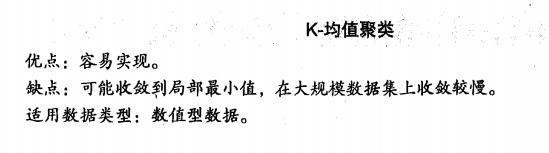K-均值是发现给定数据集的K个簇的算法。簇个数k是用户给定的，每一个簇通过质心，即簇中所有点的中心来描述。

首先，随机确定k个起始点为质心。然后将数据集中的每个点分配到一个簇中，具体来讲，为每个点找到距其最近的质心，并将其分配给该质心所对应的簇。这一步完成后，每个簇的质心更新为该簇所有点的平均值。

伪代码：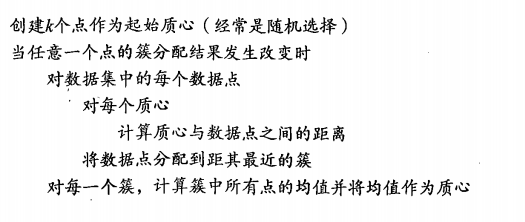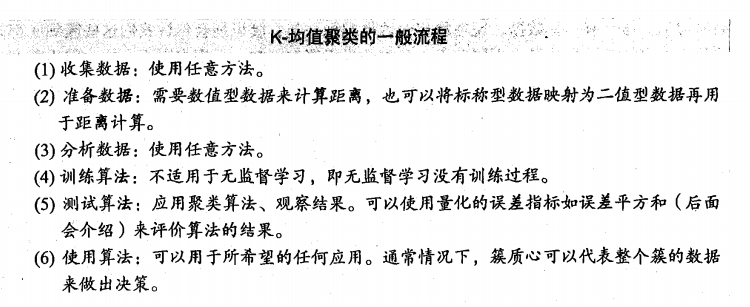下面给出K-均值算法的代码实现：

```from numpy import *

dataMat = []
fr = open(filename)
curLine = line.strip().split('\t')
fltLine = list(map(float, curLine))
#print(fltLine)
dataMat.append(fltLine)
return dataMat #返回一个包含许多列表的列表，容易将很多值封装到矩阵中

# 使用欧式距离计算两个向量的距离
def distEclud(vecA, vecB):
# 数组元素求平方
return sqrt(sum(power(vecA - vecB, 2))) #la.norm(vecA-vecB)

# 构建一个包含k个随机质心的集合
def randCent(dataSet, k):
n = shape(dataSet)
centroids = mat(zeros((k, n)))
for j in range(n):
'''
随机质心必须要整个数据集的边界之内
找到每个维的最大值和最小值，求出范围
然后生成0到1.0之间的随机数并通过最小值和取值范围，以便确保随机点在数据边界之内
'''
minJ = min(dataSet[:,j])
rangeJ = float(max(dataSet[:,j]) - minJ)
centroids[:,j] = mat(minJ + rangeJ * random.rand(k,1)) # 随机样本位于0到1中
return centroids
```

首先观察矩阵中的最大值与最小值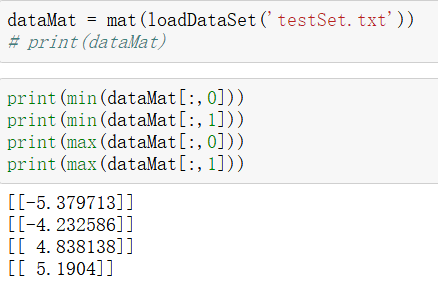然后看看randCent()函数能否生成min到max之间的值：从下图观察是可以的。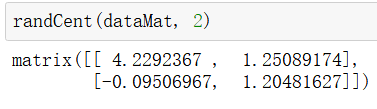再测试一下距离的计算方法：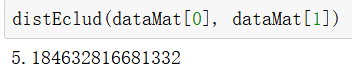所有的支持函数都可以正常运行。就可以开始实现k-均值算法了，该算法会创建k个质心，然后将每个点分配到距离最近的质心，再重新计算质心，重复数次，直到数据点的簇分配结果不再改变为止。

```def kMeans(dataSet, k, distMeas=distEclud, createCent=randCent):
'''
输入四个参数：数据集 k是必选参数，计算距离参数和创建初始质心参数是可选的
'''
m = shape(dataSet) # 数据集样本点数
# 簇分配结果矩阵 一列记录簇索引值 一列存储误差：指当前点到簇质心的距离
clusterAssment = mat(zeros((m,2)))
centroids = createCent(dataSet, k) # 创建k个质心
clusterChanged = True # 标志变量，值为true就继续迭代
while clusterChanged:
clusterChanged = False
for i in range(m):#for each data point assign it to the closest centroid
minDist = inf; minIndex = -1
for j in range(k):
distJI = distMeas(centroids[j,:],dataSet[i,:])
if distJI < minDist:
minDist = distJI; minIndex = j
if clusterAssment[i,0] != minIndex: clusterChanged = True
clusterAssment[i,:] = minIndex,minDist**2
print (centroids)
for cent in range(k):#recalculate centroids
ptsInClust = dataSet[nonzero(clusterAssment[:,0].A==cent)]#get all the point in this cluster
centroids[cent,:] = mean(ptsInClust, axis=0) # 沿矩阵列方向计算均值
return centroids, clusterAssment```

导入数据集运行结果如下：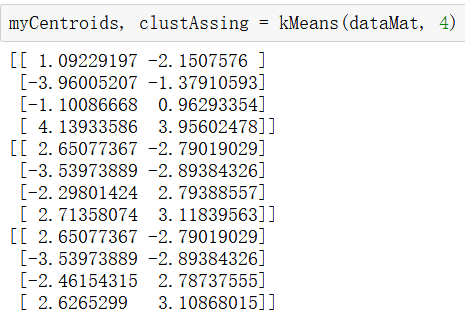上面结果给出了四个质心，可以看到经过3次迭代之后算法收敛。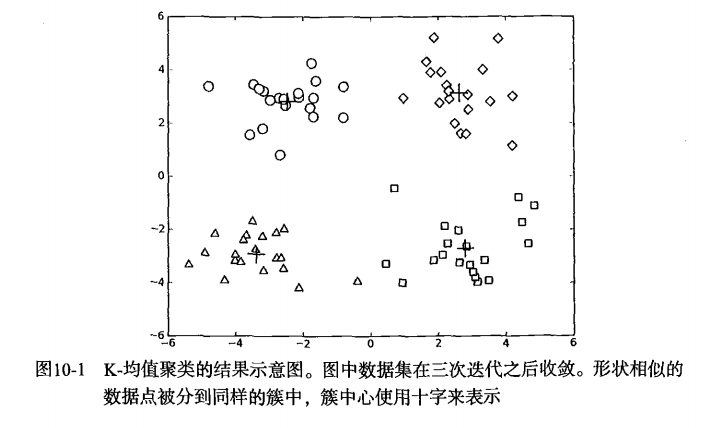## 2. 使用后处理来提高聚类性能

k是一个用户预先定义的参数，但是如何才能知道k的选择是否正确呢？如何才能知道簇的生成式比较好的呢？在包含簇分配结果的矩阵中保存着每个点的误差，即该点到簇质心的距离的平方值。我们会利用该误差来评价聚类质量的方法。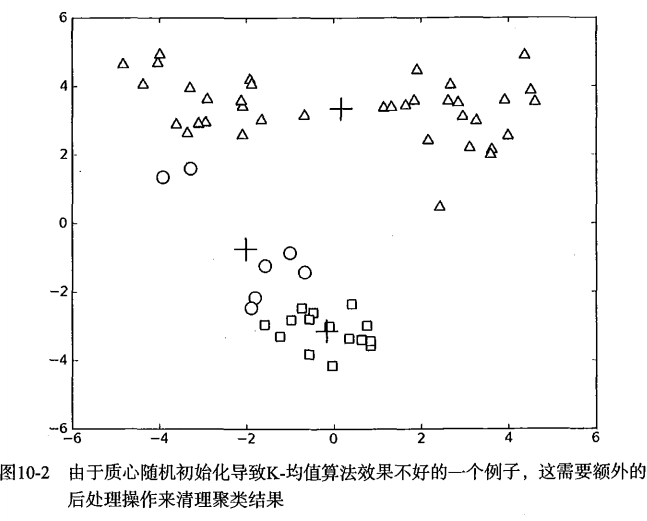图10-2的聚类结果，这是一个三个簇的数据集在运行了k均值算法之后的结果，但是点的簇分配结果并没有那么准确。k均值聚类算法比较差的原因是算法收敛到了局部最小值，而不是全局最小值。

一种度量聚类效果的指标是SSE（sum of squared error, 误差平方和），对应上面程序中clusterAssment矩阵的第一列之和。SSE值越小表示数据点越接近于它们的质心，聚类效果也就越好。因为对误差取了平方，所以更重视那些远离中心的点。

那么该怎么对10-2的结果进行改进？可以对生成的簇进行后处理，一种方法是将具有最大SSE值的簇划分成两个簇。具体实现时可以将最大簇包含的点过滤出来并在这些点上面进行k-均值算法，k=2。

为了保持簇总数不变，可以将某两个簇合并。从图中可以很明显的看出，要对下面两个出错的簇进行合并，但是我们这是在二维可视化的结果进行分析的，如果是四十维的数据应该如何处理？

有两种解决方法：合并最近的质心，或者合并两个使得SSE增幅最小的质心。第一种思路计算所有质心之间的距离，然后合并最近的两个点来实现；第二种方法需要合并两个簇然后计算总的SSE值。必须在所有可能的两个簇上重复上述的过程，直到找到合并最佳的两个簇为止。

## 3. 二分K-均值算法

为了克服k-均值算法收敛到局部最小值的问题，有人提出了另一个称为二分k-均值的算法。该算法首先将所有点作为一个簇，然后将簇一分为二。之后选择其中一个簇继续划分，选择哪一个簇进行划分取决于是否可以最大程度降低SSE的值。上述的划分福偶成不断重复，直到得到用户指定的簇数目为止。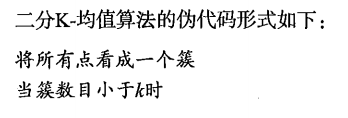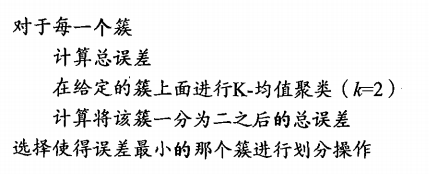另一种做法是选择SSE最大的簇进行划分，直到簇数目达到用户的指定数目，下面来实现以下实际效果：

```def biKmeans(dataSet, k, distMeas=distEclud):
m = shape(dataSet)
clusterAssment = mat(zeros((m,2))) # 存放分配结果及平方误差
centroid0 = mean(dataSet, axis=0).tolist()
centList =[centroid0] #列表 存放整个数据集的质心
for j in range(m):# 遍历数据集中所有的点来计算每个点到质心的误差值
clusterAssment[j,1] = distMeas(mat(centroid0), dataSet[j,:])**2
while (len(centList) < k):
lowestSSE = inf
for i in range(len(centList)):
ptsInCurrCluster = dataSet[nonzero(clusterAssment[:,0].A==i),:]#get the data points currently in cluster i
centroidMat, splitClustAss = kMeans(ptsInCurrCluster, 2, distMeas)
sseSplit = sum(splitClustAss[:,1])#compare the SSE to the currrent minimum
sseNotSplit = sum(clusterAssment[nonzero(clusterAssment[:,0].A!=i),1])
print ("sseSplit, and notSplit: ",sseSplit,sseNotSplit)
if (sseSplit + sseNotSplit) < lowestSSE:
bestCentToSplit = i
bestNewCents = centroidMat
bestClustAss = splitClustAss.copy()
lowestSSE = sseSplit + sseNotSplit
bestClustAss[nonzero(bestClustAss[:,0].A == 1),0] = len(centList) #change 1 to 3,4, or whatever
bestClustAss[nonzero(bestClustAss[:,0].A == 0),0] = bestCentToSplit
print ('the bestCentToSplit is: ',bestCentToSplit)
print ('the len of bestClustAss is: ', len(bestClustAss))
centList[bestCentToSplit] = bestNewCents[0,:].tolist()#replace a centroid with two best centroids
centList.append(bestNewCents[1,:].tolist())
clusterAssment[nonzero(clusterAssment[:,0].A == bestCentToSplit),:]= bestClustAss#reassign new clusters, and SSE
return mat(centList), clusterAssment```

运行结果：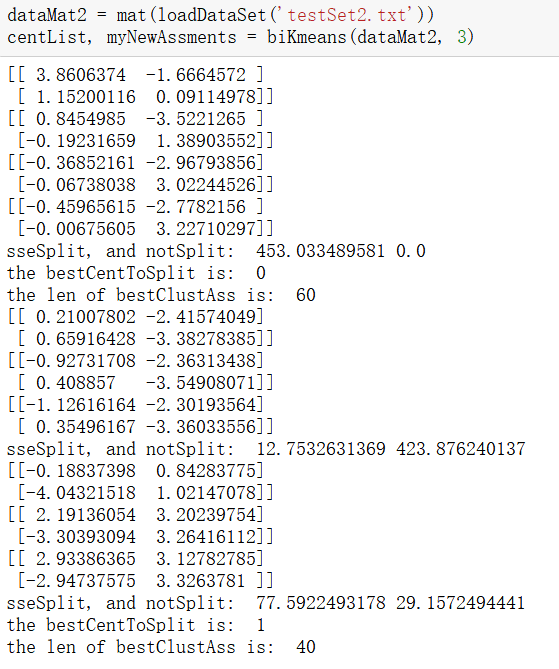质心：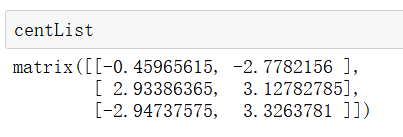上述函数可以运行多次，聚类会收敛到全局最小值，而原始的kMeans()会陷入局部最小值。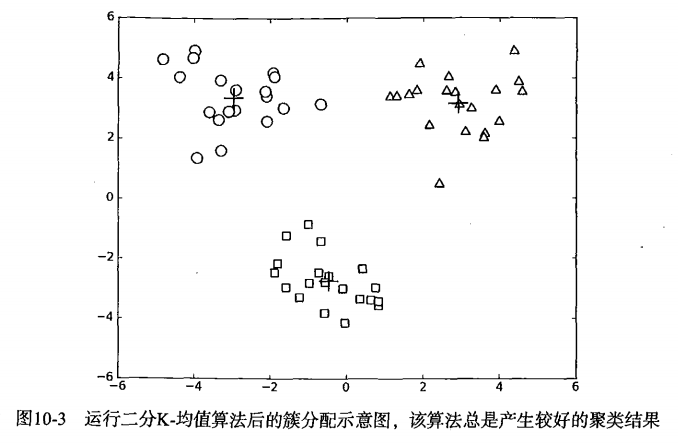## 4.本章小结

k-均值聚类是一种广泛的使用方法，以k个随机质心开始，算法会计算每个点到质心的距离，每个点会被分配到距其最近的簇质心，然后基于新分配到簇的点更新簇质心。以上过程重复数次，直到簇质心不再改变。这个方法非常简单有效，但是会受到初始簇质心的影响，为了获得更好的聚类效果可以使用另一种二分k-均值聚类算法。二分k-均值算法首先将所有点作为一个簇，然后使用k-均值聚类算法（k=2）对其划分。下一次迭代时，选择有最大误差的簇进行划分。该过程重复，直到k个簇创建成功为止。

k聚类机器学习实战 相关内容

2019-12-21 10:06:30 nanashi_F 阅读数 11
• ###### python数据分析与机器学习实战【2019新版】

购买课程后，请扫码进入学习群，获取唐宇迪老师答疑 Python数据分析与机器学习实战教程，该课程精心挑选真实的数据集为案例，通过python数据科学库numpy,pandas,matplot结合机器学习库scikit-learn完成一些列的机器学习案例。课程以实战为基础，所有课时都结合代码演示如何使用这些python库来完成一个真实的数据案例。算法与项目相结合，选择经典kaggle项目，从数据预处理开始一步步代码实战带大家入门机器学习。学完该课程即可： 1.掌握Python数据科学工具包，包括矩阵数据处理与可视化展示。 2.掌握机器学习算法原理推导，从数学上理解算法是怎么来的以及其中涉及的细节。 3.掌握每一个算法所涉及的参数，详解其中每一步对结果的影响。 4.熟练使用Python进行建模实战，基于真实数据集展开分析，一步步完成整个建模实战任务。

93869 人正在学习 去看看 唐宇迪

# 一、概述

K均值（K-means）聚类算法可以发现k个不同的簇，且每个簇的中心采用簇中所含值的均值计算而成。

• 优缺点
优点：容易实现。
缺点：可能收敛到局部最小值，在大规模数据集上收敛较慢。

• 适用数据类型
数值型数据

• K-均值聚类的一般流程
(1) 收集数据：使用任意方法。
(2) 准备数据：需要数值型数据来计算距离，也可以将标称型数据映射为二值型数据再用于距离计算。
(3) 分析数据：使用任意方法。
(4) 训练算法：不适用于无监督学习，即无监督学习没有训练过程
(5) 测试算法：应用聚类算法、观察结果。可以使用量化的误差指标如误差平方和来评价算法的结果。
(6) 使用算法：可以用于所希望的任何应用。通常情况下，簇质心可以代表整个簇的数据来做出决策。

# 二、基本概念

## 1.K-均值聚类算法

K-均值是发现给定数据集的k个簇的算法。簇个数k是用户给定的，每一个簇通过其质心（centroid），即簇中所有点的中心来描述。

K-均值算法的工作流程伪代码如下：

``````创建k个点作为起始质心（经常是随机选择）

对数据集中的每个数据点
对每个质心
计算质心与数据点之间的距离
将数据点分配到距其最近的簇

``````

## 2.使用后处理来提高聚类性能• SSE
SSE（Sum of Squared Error，误差平方和）是一种用于度量聚类效果的指标，SSE值越小表示数据点越接近于它们的质心，聚类效果也越好。因为对误差取了平方，因此更加重视那些远离中心的点。一种肯定可以降低SSE值的方法是增加簇的个数，但这违背了聚类的目标。聚类的目标是在保持簇数目不变的情况下提高簇的质量。

1. 合并最近的质心，通过计算所有质心之间的距离， 然后合并距离最近的两个点来实现。
2. 合并两个使得SSE增幅最小的质心，需要合并两个簇然后计算总SSE值。必须在所有可能的两个簇上重复上述处理过程，直到找到合并最佳的两个簇为止。

## 3.二分 K-Means 聚类算法

``````将所有点看成一个簇

计算总误差
在给定的簇上面进行 KMeans 聚类（k=2）
计算将该簇一分为二之后的总误差

``````

# 三、代码实现

## 1.K-均值聚类算法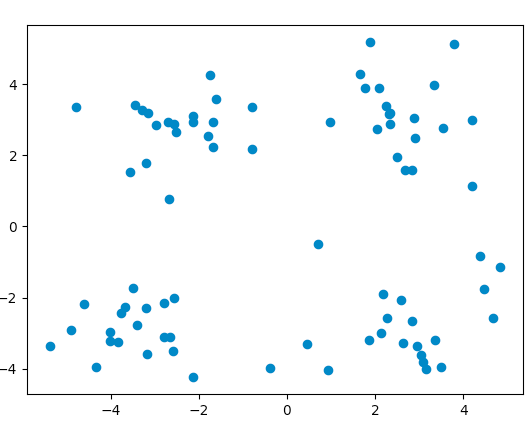``````import numpy as np
import matplotlib.pyplot as plt

"""
加载数据集
- - - -
fileName - 文件路径
"""
dataMat = []
fr = open(fileName)
curLine = line.strip().split('\t')
#映射所有的元素为 float（浮点数）类型
fltLine = list(map(float,curLine))
dataMat.append(fltLine)
return np.mat(dataMat)

def distEclud(vecA, vecB):
"""
计算两个向量的欧式距离
- - - -
vecA - 向量A

vecB - 向量B
"""
return np.sqrt(np.sum(np.power(vecA - vecB, 2)))

def randCent(dataSet, k):
"""
构建一个包含 k 个随机质心的集合
- - - -
dataSet - 数据集

k - 簇的数目
"""
# 列的数量，即数据的特征个数
n = np.shape(dataSet)
# 创建k个质心矩阵
centroids = np.mat(np.zeros((k,n)))
# 在每一维的边界内创建随机簇质心
for j in range(n):
minJ = min(dataSet[:,j])
# 范围 = 最大值 - 最小值
rangeJ = float(max(dataSet[:,j]) - minJ)
centroids[:,j] = np.mat(minJ + rangeJ * np.random.rand(k,1))
return centroids

def kMeans(dataSet, k, distMeas=distEclud, createCent=randCent):
"""
K-Means 聚类算法
- - - -
dataSet - 数据集

k - 簇的数目

distMeas - 计算距离函数

createCent - 创建初始质心函数
"""
m = np.shape(dataSet)
# 一列记录簇索引值，一列存储误差(误差是指当前点到簇质心的距离)
clusterAssment = np.mat(np.zeros((m, 2)))
centroids = createCent(dataSet, k)
# 记录簇改变
clusterChanged = True
while clusterChanged:
clusterChanged = False
# 循环每一个数据点并分配到最近的质心中去
for i in range(m):
minDist = np.inf; minIndex = -1
for j in range(k):
distJI = distMeas(centroids[j,:],dataSet[i,:])
if distJI < minDist:
minDist = distJI; minIndex = j
# 簇分配结果改变
if clusterAssment[i, 0] != minIndex:
clusterChanged = True
clusterAssment[i, :] = minIndex,minDist**2
print (centroids)
# 更新质心
for cent in range(k):
# 获取该簇中的所有点
ptsInClust = dataSet[np.nonzero(clusterAssment[:, 0].A==cent)]
# 将质心修改为簇中所有点的平均值，mean 就是求平均值的
centroids[cent,:] = np.mean(ptsInClust, axis=0)
return centroids, clusterAssment

def showData(dataMat,centroids,clust,k):
"""
数据可视化
- - - -
dataMat - 数据矩阵

centroids - 质心

clust - 簇分配结果

k - 簇的个数
"""
data = np.array(dataMat)
datanum=len(data)
for i in range(k):
cluster=[]
for j in range(datanum):
if clust[j,0]==i:
cluster.append(data[j])
plt.scatter(np.transpose(cluster), np.transpose(cluster))
cen=np.array(centroids)
plt.scatter(np.transpose(cen), np.transpose(cen),marker='+')
plt.show()

def testKMeans(k,filep):
"""
测试k-means 聚类算法
- - - -
k - 簇的个数

filep - 文件路径
"""
myCentroids, clustAssing = kMeans(dataMat,k)
showData(dataMat,myCentroids,clustAssing,k)

if __name__ == "__main__":
filepath='10.KMeans/testSet.txt'
testKMeans(4,filepath)
``````

``````[[ 0.08495263 -3.1470703 ]
[ 1.79128272 -3.49504779]
[ 0.97056461  0.12167986]
[ 2.8122667  -2.14469345]]
[[-3.19984738 -2.96423548]
[ 2.58639411 -3.57601678]
[ 0.09765693  2.86443007]
[ 3.51402856 -2.07382678]]
[[-3.38237045 -2.9473363 ]
[ 2.44798442 -3.43588358]
[-0.02298687  2.99472915]
[ 3.431003   -1.363668  ]]
[[-3.53973889 -2.89384326]
[ 2.2728545  -3.41806164]
[-0.09810934  3.03211629]
[ 3.43046378 -0.893382  ]]
[[-3.53973889 -2.89384326]
[ 2.28769    -3.23832819]
[-0.29458614  3.07335139]
[ 3.66311589  0.05552433]]
[[-3.53973889 -2.89384326]
[ 2.42776071 -3.19858565]
[-0.70059397  3.13642319]
[ 3.34275475  1.111461  ]]
[[-3.53973889 -2.89384326]
[ 2.54173689 -3.11892933]
[-1.6334182   3.03655888]
[ 3.00278739  2.19743744]]
[[-3.53973889 -2.89384326]
[ 2.65077367 -2.79019029]
[-2.46154315  2.78737555]
[ 2.6265299   3.10868015]]
``````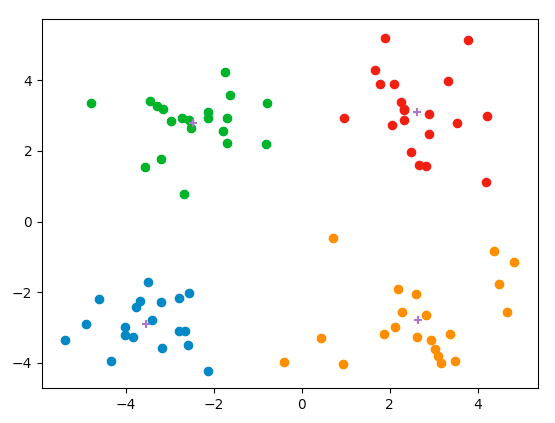## 3.二分 K-Means 聚类算法

``````def biKMeans(dataSet, k, distMeas=distEclud):
"""
二分 KMeans 聚类算法
- - - -
dataSet - 数据集

k - 簇的数目

distMeas - 计算距离函数
"""
m = np.shape(dataSet)
# 保存每个数据点的簇分配结果和平方误差
clusterAssment = np.mat(np.zeros((m,2)))
# 质心初始化为所有数据点的均值
centroid0 = np.mean(dataSet, axis=0).tolist()
# 存放质心
centList =[centroid0]
# 计算所有数据点到初始质心的距离平方误差
for j in range(m):
clusterAssment[j,1] = distMeas(np.mat(centroid0), dataSet[j,:])**2
# 当质心数量小于 k 时，二分迭代
while (len(centList) < k):
lowestSSE = np.inf
for i in range(len(centList)):
# 获取当前簇 i 下的所有数据点，进行二分 kMeans 处理
ptsInCurrCluster = dataSet[np.nonzero(clusterAssment[:,0].A==i),:]
centroidMat, splitClustAss = kMeans(ptsInCurrCluster, 2, distMeas)
# 将二分 kMeans 结果中的平方和的距离进行求和
sseSplit = np.sum(splitClustAss[:,1])
# 将未参与二分 kMeans 分配结果中的平方和的距离进行求和
sseNotSplit = np.sum(clusterAssment[np.nonzero(clusterAssment[:,0].A!=i),1])
print ("sseSplit, and notSplit: ",sseSplit,sseNotSplit)
#若总误差和小于最小误差和，记录
if (sseSplit + sseNotSplit) < lowestSSE:
bestCentToSplit = i
bestNewCents = centroidMat
bestClustAss = splitClustAss.copy()
lowestSSE = sseSplit + sseNotSplit
# 找出最好的簇分配结果
bestClustAss[np.nonzero(bestClustAss[:,0].A == 1),0] = len(centList)
bestClustAss[np.nonzero(bestClustAss[:,0].A == 0),0] = bestCentToSplit
print ('the bestCentToSplit is: ',bestCentToSplit)
print ('the len of bestClustAss is: ', len(bestClustAss))
# 更新质心列表
centList[bestCentToSplit] = bestNewCents[0,:].tolist()
centList.append(bestNewCents[1,:].tolist())
# 重新分配最好簇下的数据（质心）以及SSE
clusterAssment[np.nonzero(clusterAssment[:,0].A == bestCentToSplit),:]= bestClustAss
return np.mat(centList), clusterAssment

def testbiKMeans(k,filep):
"""
测试二分 KMeans 聚类算法
- - - -
k - 簇的个数

filep - 文件路径
"""
myCentroids, clustAssing = biKMeans(dataMat,k)
showData(dataMat,myCentroids,clustAssing,k)

if __name__ == "__main__":
#1.测试k-means 聚类算法
""" filepath='10.KMeans/testSet.txt'
testKMeans(4,filepath) """
#2.测试二分 KMeans 聚类算法
filepath='10.KMeans/testSet2.txt'
testbiKMeans(3,filepath)
``````

``````[[-3.14742611 -3.12648867]
[-4.43243513  4.42896576]]
[[-0.32150057 -2.62473743]
[-0.06953469  3.29844341]]
[[-0.45965615 -2.7782156 ]
[-0.00675605  3.22710297]]
sseSplit, and notSplit:  1216.4437240993682 0.0
the bestCentToSplit is:  0
the len of bestClustAss is:  60
[[-1.50786484 -3.48728639]
[-1.31476755 -2.92558692]]
[[-0.60498967 -3.775502  ]
[-0.43400906 -2.60222388]]
[[ 0.124341   -3.594312  ]
[-0.77411615 -2.33877908]]
[[ 0.34658275 -3.48379675]
[-0.99714875 -2.30782817]]
[[ 0.35496167 -3.36033556]
[-1.12616164 -2.30193564]]
sseSplit, and notSplit:  23.82180447123951 1216.4437240993682
[[-1.45823624  4.48218902]
[-3.14911701  1.49929085]]
[[ 0.94818419  3.40211065]
[-3.29599467  2.62429878]]
[[ 2.76275171  3.12704005]
[-3.06779095  3.33769884]]
[[ 2.93386365  3.12782785]
[-2.94737575  3.3263781 ]]
sseSplit, and notSplit:  60.59976828718332 0.0
the bestCentToSplit is:  1
the len of bestClustAss is:  40
``````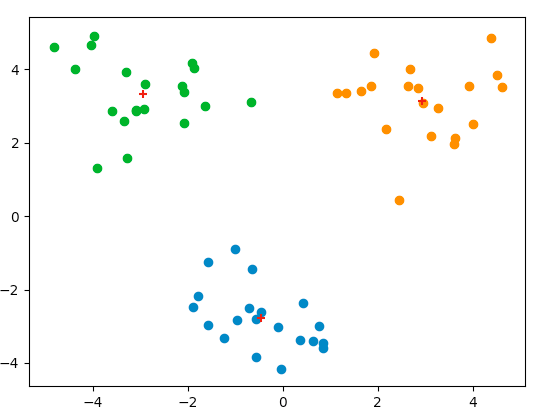# 四、小结

1. 聚类是一种无监督的学习方法。所谓无监督学习是指事先并不知道要寻找的内容，即没有目标变量。
2. 聚类将数据点归到多个簇中，其中相似数据点处于同一簇，而不相似数据点处于不同簇中。
3. 聚类中可以使用多种不同的方法来计算相似度。
一种广泛使用的聚类算法是K-均值算法，其中k是用户指定的要创建的簇的数目。
4. K-均值聚类算法以k个随机质心开始。算法会计算每个点到质心的距离。每个点会被分配到距其最近的簇质心，然后紧接着基于新分配到簇的点更新簇质心。以上过程重复数次，直到簇质心不再改变。这个简单的算法非常有效但是也容易受到初始簇质心的影响
5. 为了获得更好的聚类效果，可以使用另一种称为二分K-均值的聚类算法。
二分K-均值算法首先将所有点作为一个簇，然后使用K均值算法（k = 2）对其划分。下一次迭代时，选择有最大误差的簇进行划分。该过程重复直到k个簇创建成功为止。二分K-均值的聚类效果要好于K-均值算法。

k聚类机器学习实战 相关内容

2018-07-11 16:27:41 weixin_42314808 阅读数 820
• ###### python数据分析与机器学习实战【2019新版】

购买课程后，请扫码进入学习群，获取唐宇迪老师答疑 Python数据分析与机器学习实战教程，该课程精心挑选真实的数据集为案例，通过python数据科学库numpy,pandas,matplot结合机器学习库scikit-learn完成一些列的机器学习案例。课程以实战为基础，所有课时都结合代码演示如何使用这些python库来完成一个真实的数据案例。算法与项目相结合，选择经典kaggle项目，从数据预处理开始一步步代码实战带大家入门机器学习。学完该课程即可： 1.掌握Python数据科学工具包，包括矩阵数据处理与可视化展示。 2.掌握机器学习算法原理推导，从数学上理解算法是怎么来的以及其中涉及的细节。 3.掌握每一个算法所涉及的参数，详解其中每一步对结果的影响。 4.熟练使用Python进行建模实战，基于真实数据集展开分析，一步步完成整个建模实战任务。

93869 人正在学习 去看看 唐宇迪

# 机器学习实战—K-means聚类

## K-means聚类算法原理及其相关概念

##### 1.欧式距离（edclidean distance）：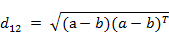##### 2.曼哈顿距离（Manhattan distance）：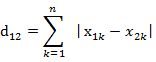##### 3.马氏距离（mahalanobis distance）：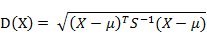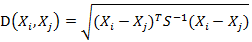## K-means聚类算法

对数据集中的每个点：

对每个质心：

计算质心与数据点之间的距离

将数据点分配到距离其最近的簇

对每一个簇，计算簇中所有的点的均值并将均值作为质心。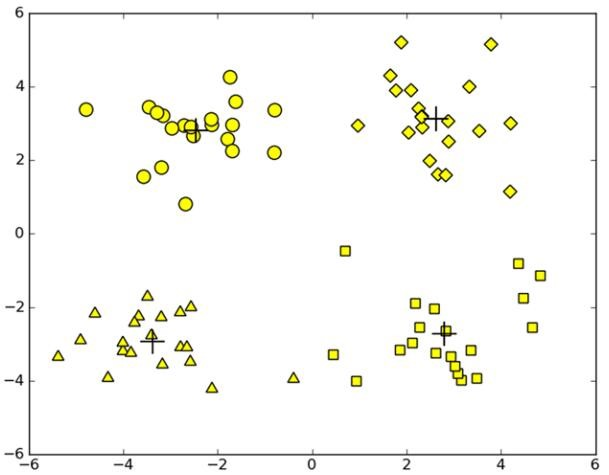## 聚类算法的缺陷及如何提高聚类性能

2.k-均值算法收敛到局部最小值，而不是全局最小值提高聚类性能：由于执行随机初始化导致K-均值收敛效果较差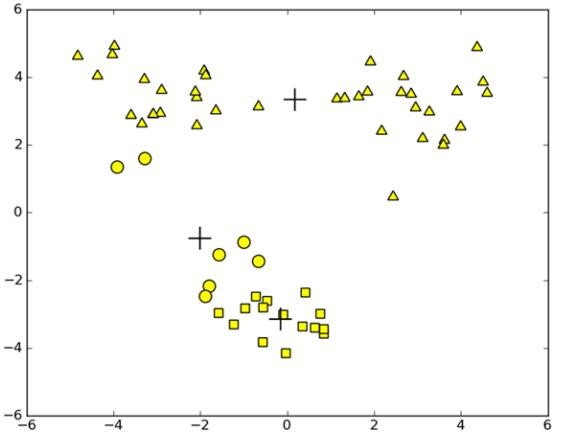2、遍历簇合并两个然后计算SSE的值，找到使得SSE最小的情况。

## 二分K-均值算法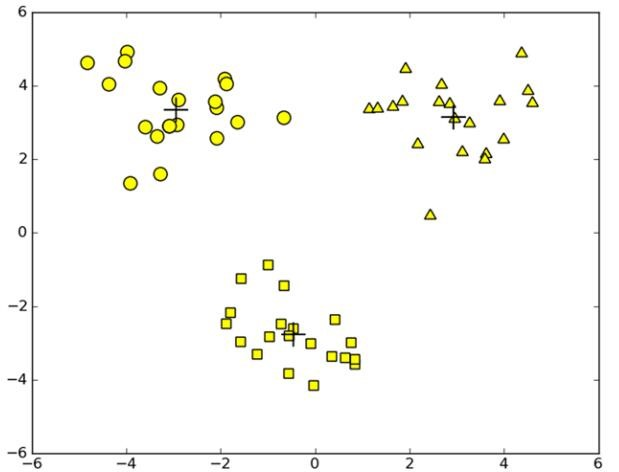## 代码：

```from numpy import *

dataMat = []
fr = open(fileName)
for line in fr.readlines(): #for each line
curLine = line.strip().split('\t')
fltLine = list(map(float,curLine)) #这里和书中不同 和上一章一样修改
dataMat.append(fltLine)
return dataMat

#distance func
def distEclud(vecA,vecB):
return sqrt(sum(power(vecA - vecB, 2)))  # la.norm(vecA-vecB) 向量AB的欧式距离

#init K points randomly
def randCent(dataSet, k):
n = shape(dataSet)
centroids = mat(zeros((k,n)))#create centroid mat
for j in range(n):#create random cluster centers, within bounds of each dimension
minJ = min(dataSet[:,j])
rangeJ = float(max(dataSet[:,j]) - minJ)
centroids[:,j] = mat(minJ + rangeJ * random.rand(k,1))
return centroids

#K-均值算法:
def kMeans(dataSet,k,distMeas=distEclud,createCent=randCent):
#参数：dataset,num of cluster,distance func,initCen
m=shape(dataSet)
clusterAssment=mat(zeros((m,2)))#store the result matrix,2 cols for index and error
centroids=createCent(dataSet,k)
clusterChanged=True
while clusterChanged:
clusterChanged=False
for i in range(m):#for every points
minDist = inf;minIndex = -1#init
for j in range(k):#for every k centers，find the nearest center
distJI=distMeas(centroids[j,:],dataSet[i,:])
if distJI<minDist:#if distance is shorter than minDist
minDist=distJI;minIndex=j# update distance and index(类别)
if clusterAssment[i,0] != minIndex:
clusterChanged = True
#此处判断数据点所属类别与之前是否相同（是否变化，只要有一个点变化就重设为True，再次迭代）
clusterAssment[i,:] = minIndex,minDist**2
#print(centroids)
# update k center
for cent in range(k):
ptsInClust=dataSet[nonzero(clusterAssment[:,0].A==cent)]
centroids[cent,:]=mean(ptsInClust,axis=0)
return centroids,clusterAssment

#二分K-均值聚类
def biKmeans(dataSet,k,distMeas=distEclud):
m=shape(dataSet)
clusterAssment=mat(zeros((m,2)))
centroid0 = mean(dataSet, axis=0).tolist()
centList = [centroid0]  # create a list with one centroid
for j in range(m):  # calc initial Error for each point
clusterAssment[j, 1] = distMeas(mat(centroid0), dataSet[j, :]) ** 2
while (len(centList) < k):
lowestSSE = inf #init SSE
for i in range(len(centList)):#for every centroid
ptsInCurrCluster = dataSet[nonzero(clusterAssment[:, 0].A == i),:]  # get the data points currently in cluster i
centroidMat, splitClustAss = kMeans(ptsInCurrCluster, 2, distMeas)# k=2,kMeans
sseSplit = sum(splitClustAss[:, 1])  # compare the SSE to the currrent minimum
sseNotSplit = sum(clusterAssment[nonzero(clusterAssment[:, 0].A != i), 1])
print("sseSplit, and notSplit: ", sseSplit, sseNotSplit)
if (sseSplit + sseNotSplit) < lowestSSE: #judge the error
bestCentToSplit = i
bestNewCents = centroidMat
bestClustAss = splitClustAss.copy()
lowestSSE = sseSplit + sseNotSplit
#new cluster and split cluster
bestClustAss[nonzero(bestClustAss[:, 0].A == 1), 0] = len(centList)  # change 1 to 3,4, or whatever
bestClustAss[nonzero(bestClustAss[:, 0].A == 0), 0] = bestCentToSplit
print('the bestCentToSplit is: ', bestCentToSplit)
print('the len of bestClustAss is: ', len(bestClustAss))
centList[bestCentToSplit] = bestNewCents[0, :].tolist()  # replace a centroid with two best centroids
centList.append(bestNewCents[1, :].tolist())
clusterAssment[nonzero(clusterAssment[:, 0].A == bestCentToSplit),:] = bestClustAss  # reassign new clusters, and SSE
return mat(centList), clusterAssment

#practice example
#distance calc function：结合两个点经纬度（用角度做单位），返回地球表面两点之间距离
def distSLC(vecA, vecB):#Spherical Law of Cosines
a = sin(vecA[0,1]*pi/180) * sin(vecB[0,1]*pi/180)
b = cos(vecA[0,1]*pi/180) * cos(vecB[0,1]*pi/180) * cos(pi * (vecB[0,0]-vecA[0,0]) /180)
return arccos(a + b)*6371.0 #pi is imported with numpy

#draw function
import matplotlib
import matplotlib.pyplot as plt
def clusterClubs(numClust=5):#参数：希望得到的簇数目
datList = []
lineArr = line.split('\t')
datList.append([float(lineArr), float(lineArr)])#逐个获取第四列和第五列的经纬度信息
datMat = mat(datList)
myCentroids, clustAssing = biKmeans(datMat, numClust, distMeas=distSLC)
#draw
fig = plt.figure()
rect=[0.1,0.1,0.8,0.8]#创建矩形
#创建不同标记图案
scatterMarkers=['s', 'o', '^', '8', 'p', \
'd', 'v', 'h', '>', '<']
axprops = dict(xticks=[], yticks=[])
ax0.imshow(imgP)
for i in range(numClust):
ptsInCurrCluster = datMat[nonzero(clustAssing[:,0].A==i),:]
markerStyle = scatterMarkers[i % len(scatterMarkers)]
ax1.scatter(ptsInCurrCluster[:,0].flatten().A, ptsInCurrCluster[:,1].flatten().A, marker=markerStyle, s=90)
ax1.scatter(myCentroids[:,0].flatten().A, myCentroids[:,1].flatten().A, marker='+', s=300)
plt.show()```

## 参考文献

【1】Peter Harrington, Machine Learning inAction[M] . US 2007

k聚类机器学习实战 相关内容